＃ 卷积神经网络：逐步实现

Notation:

• 上标 $[l]$表示$l ^ {th}$图层的一个对象。
• 示例：$a ^ {}$$4 ^ {th}$层激活。 $W ^ {}$$b ^ {}$$5 ^ {th}$层参数。
• 上标$（i）$表示来自$i ^ {th}$示例的对象。
• 示例：$x ^ {（i）}$$i ^ {th}$训练示例输入。
• 下标$i$表示向量的$i ^ {th}$条目。
例如：$a ^ {[l]} _ i$表示层$l$中激活的$i ^ {th}$条目，假设这是一个完全连接（FC）层。
• $n_H$$n_W$$n_C$分别表示给定层的高度，宽度和通道数量。如果你想引用一个特定的图层$l$，你也可以编写$n_H ^ {[l]}$$n_W ^ {[l]}$$n_C ^ {[l]}$
• $n_ {H_ {prev}}$$n_ {W_ {prev}}$$n_ {C_ {prev}}$分别表示上一层的高度，宽度和通道数量。如果引用特定层$l$，这也可以表示为$n_H ^ {[l-1]}$$n_W ^ {[l-1]}$$n_C ^ {[l-1]}$

## 1 - Packages

• numpy i是用Python进行科学计算的基础包。
• matplotlib 是一个用Python绘制图表的库。
• np.random.seed（1）用于保持所有随机函数调用一致。它会帮助我们为你的工作评分。
d:\mysites\deeplearning.ai-master\.env\lib\site-packages\h5py\__init__.py:36: FutureWarning: Conversion of the second argument of issubdtype from float to np.floating is deprecated. In future, it will be treated as np.float64 == np.dtype(float).type.
from ._conv import register_converters as _register_converters


## 2 - Outline of the Assignment

• Convolution functions, including:
• Convolve window
• Convolution forward
• Convolution backward (optional)
• Pooling functions, including:
• Pooling forward
• Distribute value
• Pooling backward (optional)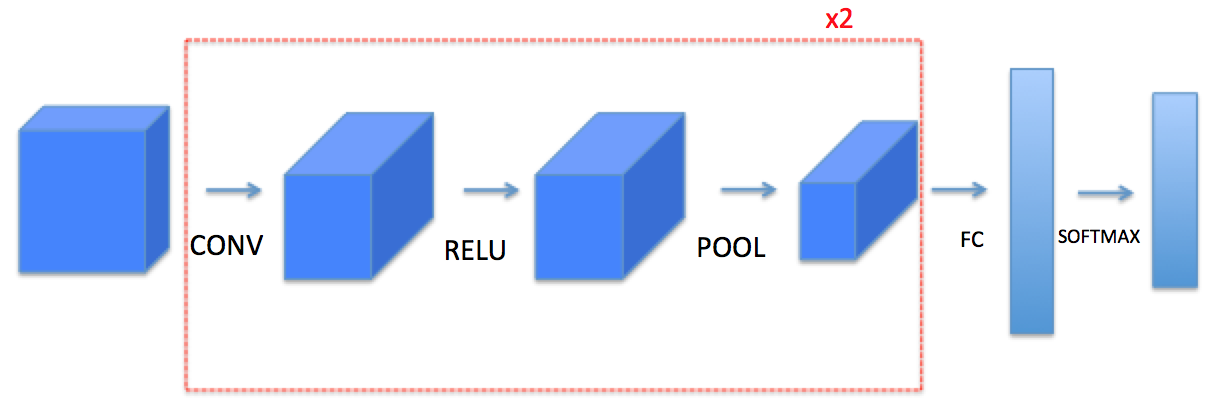## 3 - Convolutional Neural Networks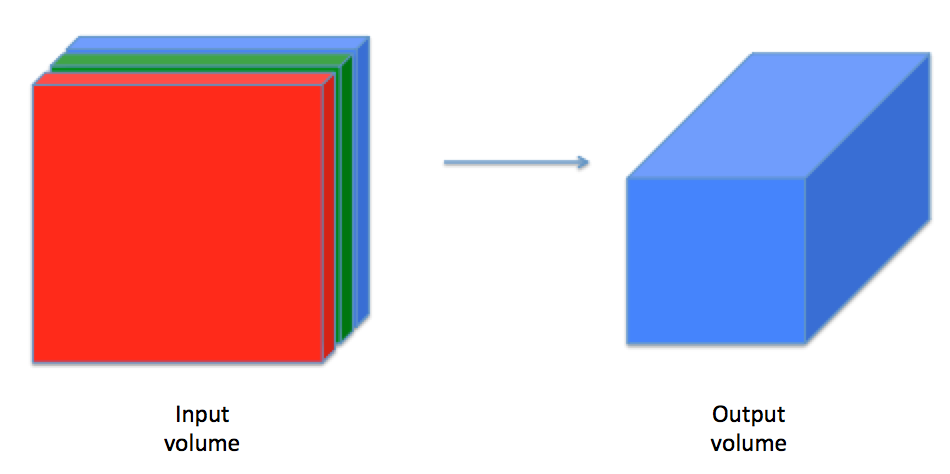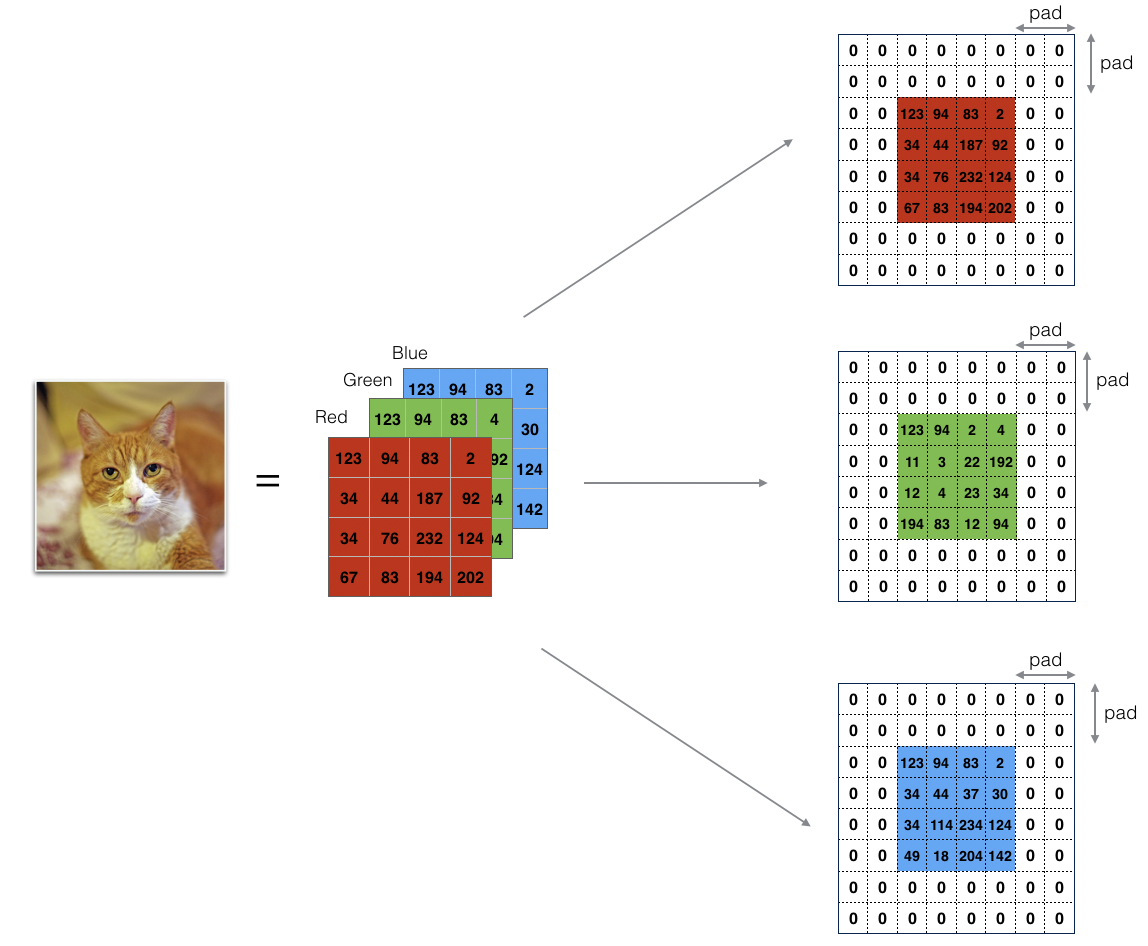Image (3 channels, RGB) with a padding of 2.

• 它允许您使用CONV层，而不必缩小卷的高度和宽度。这对于建立更深的网络非常重要，否则当你走向更深层时，高度/宽度会缩小。一个重要的特例是“相同”卷积，其中高度/宽度在一层之后被完全保留。

• 它可以帮助我们在图像边界保留更多信息。如果没有填充，下一层的极少数值将受到像素边缘的影响。

x.shape = (4, 3, 3, 2)
x_pad.shape = (4, 7, 7, 2)
x[1,1] = [[ 0.90085595 -0.68372786]
[-0.12289023 -0.93576943]
[-0.26788808  0.53035547]]
[0. 0.]
[0. 0.]
[0. 0.]
[0. 0.]
[0. 0.]
[0. 0.]]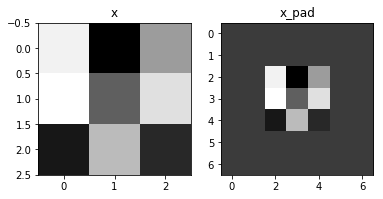Expected Output:

 x.shape: (4, 3, 3, 2) x_pad.shape: (4, 7, 7, 2) x[1,1]: [[ 0.90085595 -0.68372786] [-0.12289023 -0.93576943] [-0.26788808 0.53035547]] x_pad[1,1]: [[ 0. 0.] [ 0. 0.] [ 0. 0.] [ 0. 0.] [ 0. 0.] [ 0. 0.] [ 0. 0.]]

### 3.2 - Single step of convolution

• Takes an input volume
• Applies a filter at every position of the input
• Outputs another volume (usually of different size)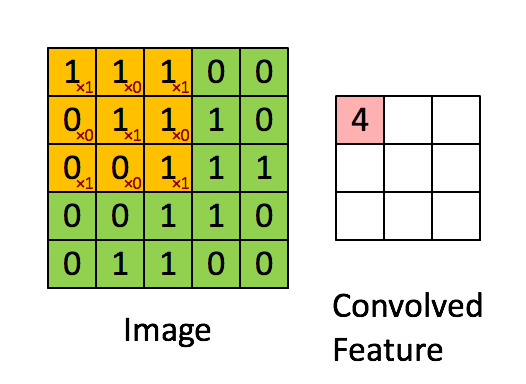Figure 2 : Convolution operation
with a filter of 2x2 and a stride of 1 (stride = amount you move the window each time you slide)

Later in this notebook, you’ll apply this function to multiple positions of the input to implement the full convolutional operation.

Exercise: Implement conv_single_step(). Hint.

Z = -6.999089450680221


Expected Output:

 Z -6.99909

### 3.3 - Convolutional Neural Networks - 正向传播

Hint:

1. 要选择矩阵“a_prev”（形状（5,5,3））左上角的2x2切片，您可以:

2.要定义a_slice，您需要首先定义它的顶点vert_start，vert_end，horiz_start和horiz_end。这个数字可能有助于您找到如何在下面的代码中使用h，w，f和s来定义每个角点。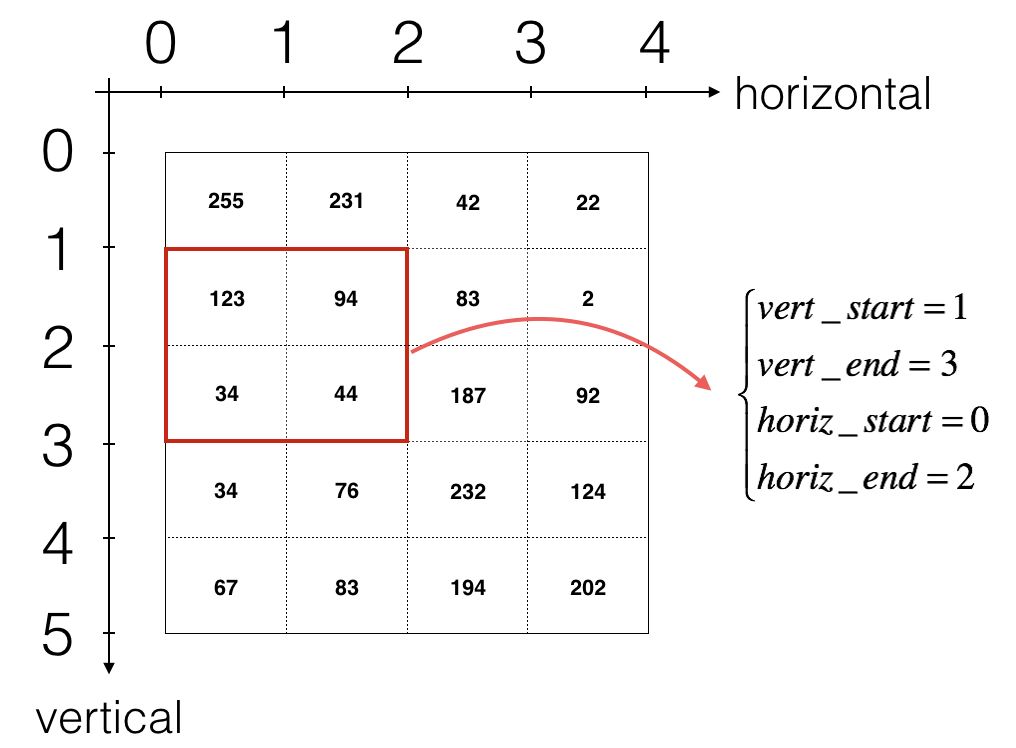Figure 3 : Definition of a slice using vertical and horizontal start/end (with a 2x2 filter)
This figure shows only a single channel.

Reminder:

$$n_H = \lfloor \frac{n_{H_{prev}} - f + 2 \times pad}{stride} \rfloor +1$$
$$n_W = \lfloor \frac{n_{W_{prev}} - f + 2 \times pad}{stride} \rfloor +1$$
$$n_C = \text{number of filters used in the convolution}$$

Z's mean = 0.004786321537477471
Z[3,2,1] = [ 0.10709871 -0.03102354 -0.52995452  0.98611224  0.65733641 -0.84239368
-0.04608241  0.08802027]
cache_conv = [-0.20075807  0.18656139  0.41005165]
(10, 4, 4, 8)


 Z’s mean 0.0489952035289 Z[3,2,1] [-0.61490741 -6.7439236 -2.55153897 1.75698377 3.56208902 0.53036437 5.18531798 8.75898442] cache_conv [-0.20075807 0.18656139 0.41005165]

## 4 - Pooling layer

• 最大池化层：在输入上滑动（$f，f$）窗口并将窗口的最大值存储在输出中。

• 平均池图层：在输入上滑动（$f，f$）窗口并在输出中存储窗口的平均值。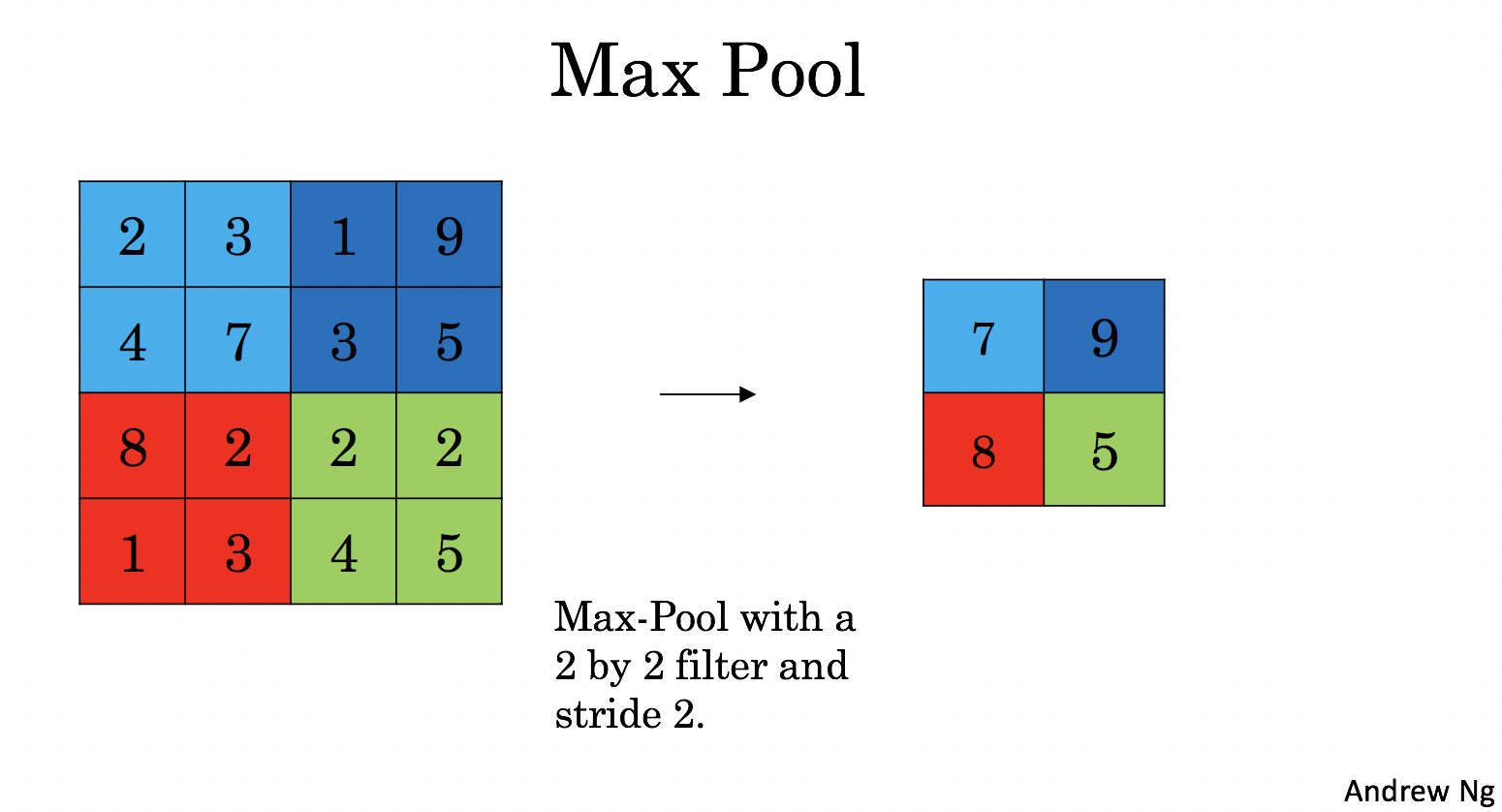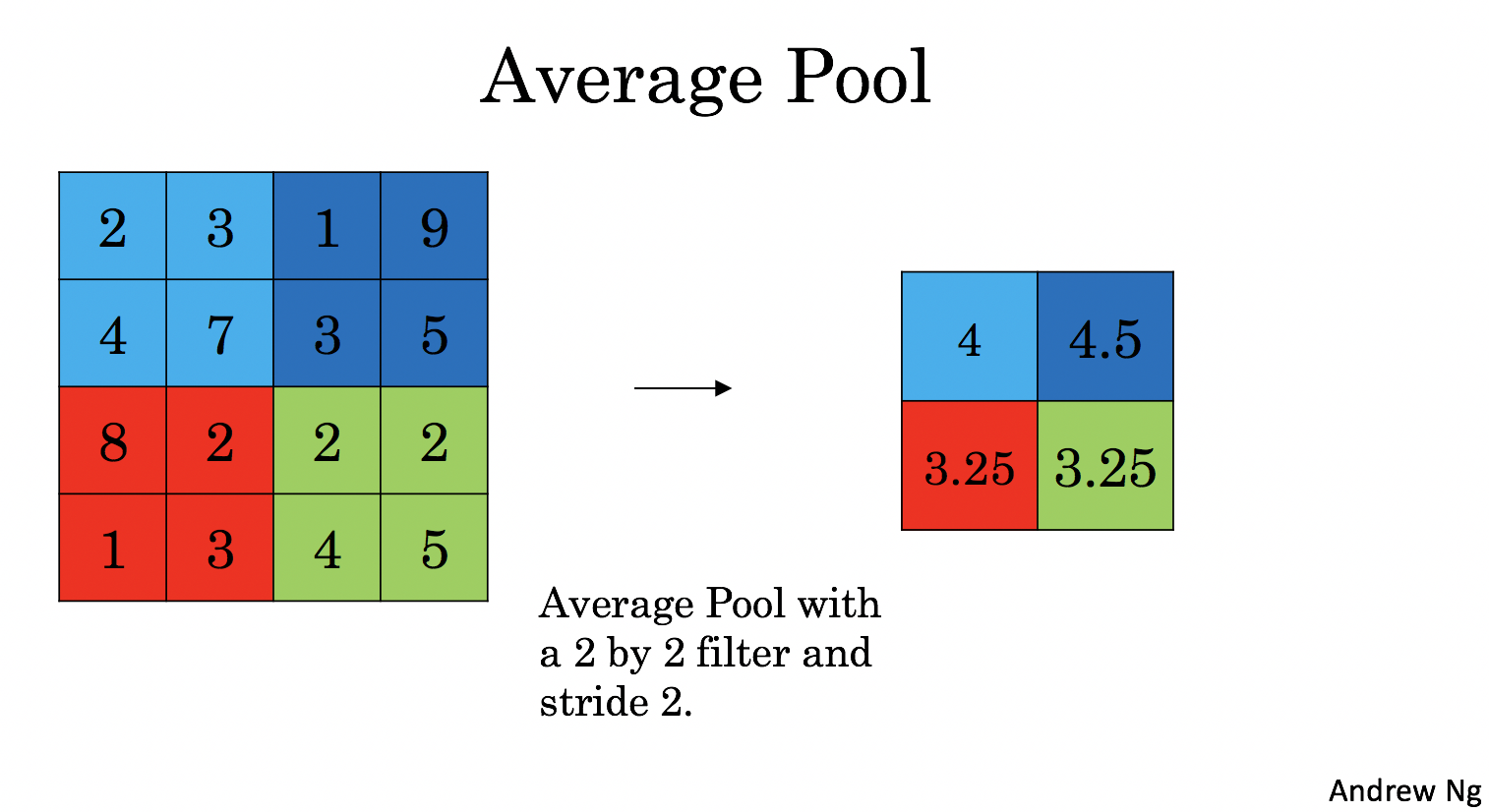mode = max
A = [[[[1.62434536 0.86540763 2.18557541]]]

[[[1.62434536 0.86540763 2.18557541]]]]

mode = average
A = [[[[ 0.24481813 -0.47568152  0.3263877 ]]]

[[[ 0.24481813 -0.47568152  0.3263877 ]]]]


 A = [[[[ 1.74481176 0.86540763 1.13376944]]] [[[ 1.13162939 1.51981682 2.18557541]]]] A = [[[[ 0.02105773 -0.20328806 -0.40389855]]] [[[-0.22154621 0.51716526 0.48155844]]]]

## 5 - 卷积神经网络中的反向传播

### 5.1 - 卷积层反向传播

#### 5.1.1 - Computing dA:

$$dA += \sum _{h=0} ^{n_H} \sum_{w=0} ^{n_W} W_c \times dZ_{hw} \tag{1}$$

In code, inside the appropriate for-loops, this formula translates into:

#### 5.1.2 - Computing dW:

$$dW_c += \sum _{h=0} ^{n_H} \sum_{w=0} ^ {n_W} a_{slice} \times dZ_{hw} \tag{2}$$

$a_ {slice}$对应于用于生成活动 {ij} $的切片。因此，这最终为我们提供了相对于该切片的$ W $的渐变。既然它是相同的$ W $，我们将只加起来所有这样的渐变来获得$ dW $。 在代码中，在适当的for循环中，该公式转换为： #### 5.1.3 - Computing db: 这是计算$db$相对于特定过滤器$W_c\$的成本的公式：

$$db = \sum_h \sum_w dZ_{hw} \tag{3}$$

dA_mean = -0.775945386961
dW_mean = 2.74605132882
db_mean = 0.765811445996


Expected Output:

 dA_mean 1.45244 dW_mean 1.72699 db_mean 7.83923

## 5.2 Pooling layer - backward pass

### 5.2.1 Max pooling - backward pass

$$X = \begin{bmatrix} 1 && 3 \\ 4 && 2 \end{bmatrix} \quad \rightarrow \quad M =\begin{bmatrix} 0 && 0 \\ 1 && 0 \end{bmatrix}\tag{4}$$

x =  [[ 1.62434536 -0.61175641 -0.52817175]
[-1.07296862  0.86540763 -2.3015387 ]]
mask =  [[ True False False]
[False False False]]


Expected Output:

 x = [[ 1.62434536 -0.61175641 -0.52817175] [-1.07296862 0.86540763 -2.3015387 ]] mask = [[ True False False] [False False False]]

### 5.2.2 - Average pooling - backward pass

$$dZ = 1 \quad \rightarrow \quad dZ =\begin{bmatrix} 1/4 && 1/4 \\ 1/4 && 1/4 \end{bmatrix}\tag{5}$$

distributed value = [[ 0.5  0.5]
[ 0.5  0.5]]


Expected Output:

 distributed_value = [[ 0.5 0.5]
[ 0.5 0.5]]

### 5.2.3 Putting it together: Pooling backward

mode = max
mean of dA =  0.145713902729
dA_prev[1,1] =  [[  0.           0.        ]
[ 10.11330283  -0.49726956]
[  0.           0.        ]]

mode = average
mean of dA =  0.145713902729
dA_prev[1,1] =  [[ 2.59843096 -0.27835778]
[ 7.96018612 -1.95394424]
[ 5.36175516 -1.67558646]]
`

Expected Output:

mode = max:

 mean of dA = 0.145713902729 dA_prev[1,1] = [[ 0. 0. ] [ 5.05844394 -1.68282702] [ 0. 0. ]]

mode = average

 mean of dA = 0.145713902729 dA_prev[1,1] = [[ 0.08485462 0.2787552 ] [ 1.26461098 -0.25749373] [ 1.17975636 -0.53624893]]